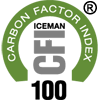# Carbon Factor Index

### ICEMAN Carbon Factor Index®

The Carbon Factor Index is a mechanism for the mathematical conversion of the Carbon Factor into a unit of measurement between 1 and 100 that represents the Carbon Factor as a percentage to carbon neutral where carbon neutral equals 100. For example a Carbon Factor Index of 80 (CFI 80) will indicate a carbon neutral measurement of 80% carbon neutral. A Carbon Factor Index of 100 (CFI 100) is 100% carbon neutral. The mathematical formulas used to convert the Carbon Factor to the Carbon Factor Index will remain proprietary and will be verified by a board of science and research. The Carbon Factor Index calculations and units may differ from one industry to another however the mathematical formulas will be standardized within each industry to maintain continuity. Baselines will be established by industry in order to determine a percentage to carbon neutral. Carbon Factor Index values in excess of 100 are possible to indicate carbon reduction programs that result in measurements in the reduction or offset of greenhouse gases greater than what is required to be carbon neutral.Frank Dalene